Friday, December 1, 2023
HomeAIWhat is an AI algorithm?

# What is an AI algorithm?

AI algorithms are constantly advancing, and new papers and services are being published every day.

On the other hand, some systems that are currently called “AI” actually run on classical algorithms. Many of them are based on old-fashioned statistical methods.

In this article, I will list representative algorithms of AI and introduce the basic algorithm mechanism.

• What is an AI algorithm?
• Basic structure of the algorithm
• Algorithms for supervised learning
• Regression and classification
• regression analysis
• k-nearest neighbor method
• random forest
• support vector machine
• Algorithms for unsupervised learning
• clustering
• k-means method
• Reinforcement learning algorithm
• Q-learning
• Other reinforcement learning algorithms
• summary

## What is an AI algorithm?

The word ” algorithm ” is difficult to describe in one sentence. Above all, there is also no proper translation in Japanese.

When the word ” algorithm ” is commonly used, it refers to some procedure. Algorithm in AI is also close to its understanding, and in short, it refers to “calculation procedure”.

### Basic structure of the algorithm

The most common algorithm is “sort”. For example, let’s consider the problem “Sort the horizontally aligned numbers in ascending order”.There are various ways to solve the problem and ways of thinking about it, but let’s simply check the magnitude relationship of the numbers from the left.

If the number on the right is smaller than the number on the left, the left-right relationship is flipped. Repeat this process until the number on the right is greater than the number on the left.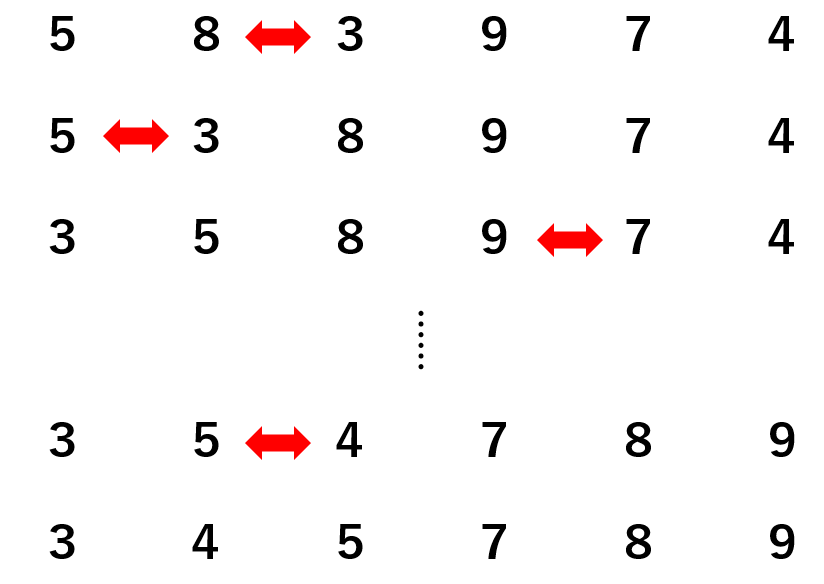This procedure is the procedure of calculation, that is, the algorithm. Did you get an image of the algorithm?

By implementing an algorithm programmatically, anyone with that program can use that algorithm.

Algorithms in AI are similar, and even if we don’t know how the algorithm works or how it is mathematically designed, we can get results just by using that algorithm. .

This is because algorithms implemented in programming are in the form of “functions”. A programmatic function is something that transforms input into output.

It’s perfectly fine for us to use the program without knowing how the functions work internally.

But understanding how algorithms work can give us a better understanding of AI itself. In this article, I will introduce various algorithms, but for the sake of intuitiveness, I will try to avoid mathematical explanations.

## Algorithms for supervised learning

### Regression and classification

Supervised learning methods can be broadly divided into regression and classification. Regression techniques deal with the problem of “predicting future numbers” for some data, while classification techniques deal with the problem of “predicting which class some data belongs to”.

In other words, regression techniques deal with ‘continuous values’, whereas classification techniques deal with ‘discrete values’. The figure below shows the difference between regression and classification.

### regression analysis

Regression analysis predicts the target variable you want to predict based on various other explanatory variables.

When there is only one explanatory variable, it is called simple regression analysis. By interpreting the objective variable y as the dependent variable and the explanatory variable x as the independent variable, simple regression analysis can be expressed as a linear function of the form “y=ax+b” with a and b as parameters. When there are multiple explanatory variables, it is called multiple regression analysis.Source: https://en.wikipedia.org/wiki/Linear_regression

There is a distinction between “linear regression” and “nonlinear regression” in regression analysis. This is an intuitive explanation that lacks rigor, but a regression analysis that can linearly express the relationship shown in the figure above, in other words, the relationship between data is called “linear regression.”

### k-nearest neighbor method

A typical classification problem algorithm is “k-nearest neighbor”. It determines to which class unknown data belongs to class-divided data scattered on coordinates.

Extract k pieces of data from the unknown data in descending order of distance, and sort the unknown data into the class with the largest number among the k pieces of data. The diagram is as follows.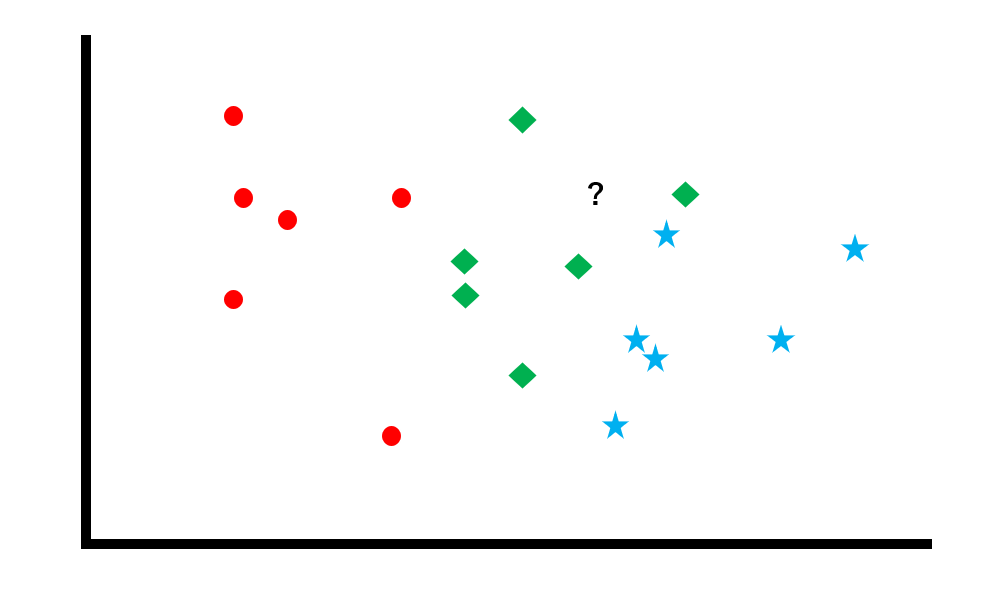Determine to which class the unknown data belongs to the already labeled data group. In this example, there are three classes: the red circle class, the blue star class, and the green diamond class.

Next, with k=3, three data are extracted from the unknown data in descending order of distance. In this example, there are 1 blue star and 2 green diamonds, so a majority vote is taken to determine that this unknown data belongs to the green diamond class.

### random forest

A random forest is a combination of several algorithms called “ decision trees ”. It may be easier to understand what a decision tree is by expressing it in a flow chart as shown below.

The image above shows a decision tree with YES/NO answers to questions.

Random forest refers to an algorithm that arranges multiple decision trees and decides the result by majority vote.Also, since there are two types of decision trees: regression decision trees and classification decision trees, random forests can handle both problems.

### support vector machine

A support vector machine is an algorithm that calculates “margin maximization” for a data group. Let’s follow the process with reference to the diagram.

Let’s consider the problem of separating red circles and blue stars from scattered data with a “boundary line”. However, as you can see in this figure, there are many ways to draw the line.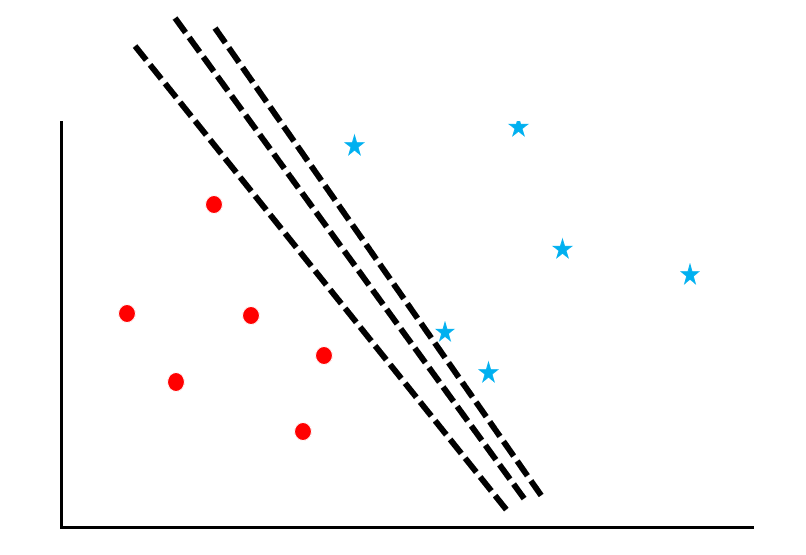Now consider “maximizing the support vector margin”. Support vectors refer to the data near the border, and margin refers to the distance between the border and the data. The green line in the figure is the margin.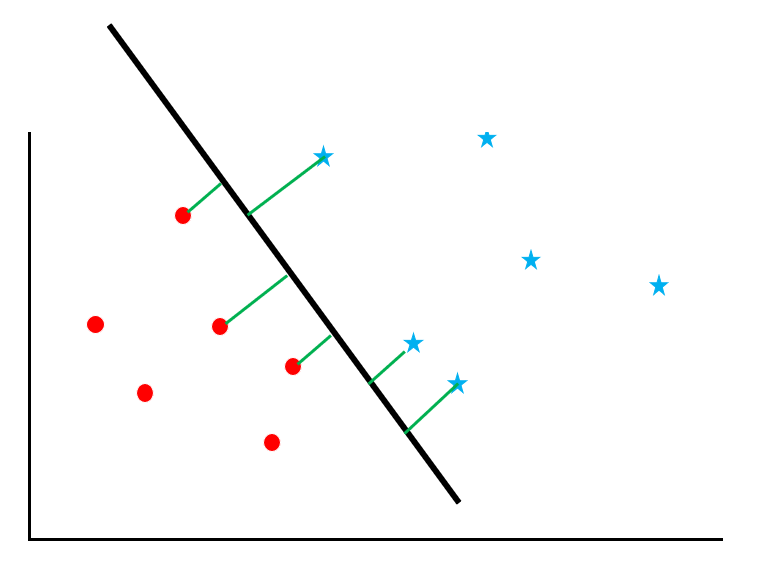The line that maximizes this margin is taken as the boundary line. This way you can avoid “false positives”. This is because maximizing the margin reduces the number of data that are ambiguous as to which of the two classes they belong to.

This support vector machine is an algorithm that can be used for both regression and classification problems.

## Algorithms for unsupervised learning

### clustering

A typical unsupervised learning algorithm is ” clustering “.

Clustering is an algorithm for grouping unknown data. The difference from the so-called classification ( supervised learning ) algorithm can be expressed as shown in the figure below.

### k-means method

The k-means method is the most commonly used clustering algorithm.

First, randomly determine k centroid points for the scattered data group and use them as the core.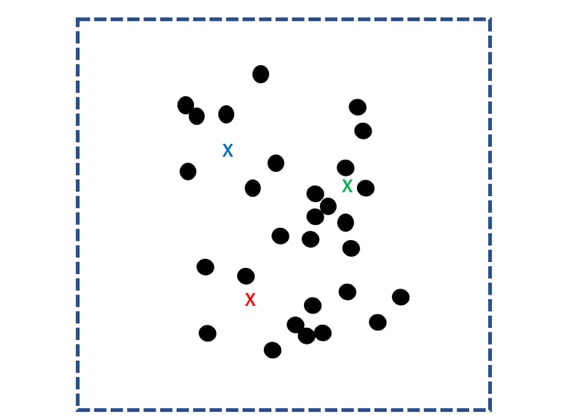Then, the distances to the k nuclei are calculated for all data and grouped into the closest nuclei. This group is called a “cluster”.Next, find the center of gravity for each cluster and use it as the new k kernels. Repeat the same process to separate each data into the nearest centroid clusters.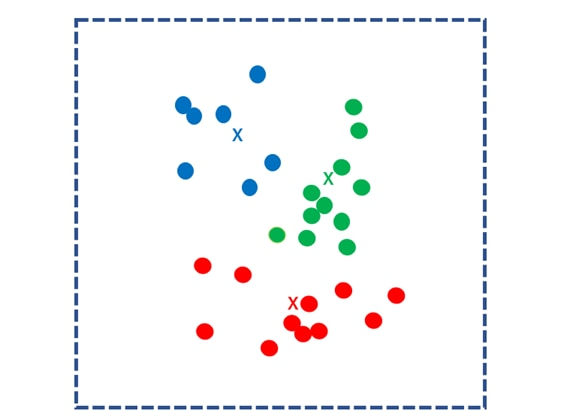Repeat this process until the center of mass no longer moves. The calculation ends when the centroid point is no longer updated.

## Reinforcement learning algorithm

### Q-learning

Q-learning is an “algorithm that learns the Q value”. Understanding mathematical formulas is an unavoidable part of learning Q-learning, but here I will try to simplify it as much as possible.

Q-learning can be expressed by the following formula.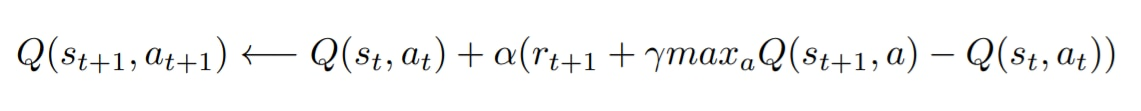This algorithm can be interpreted as “choose the action a that maximizes the reward r in the state s”.

The expected value of the reward that can be obtained by taking that action is expressed as the Q value. Since the current state s is created as a result of accumulating the value of past actions, the current state s always has a Q value. And you can update the Q value depending on what action you take next. Choosing the action with the highest Q value increases the chances of reaching the reward.

There are two types of parameters, α and γ. α is the “learning rate”, which determines how quickly the Q value is updated. γ is the “discount rate” and represents how much we can trust the Q-value of the next action to incorporate it into the current Q-value Optimizing this will result in proper learning.

### Other reinforcement learning algorithms

Other reinforcement learning algorithms include Monte Carlo and SARSA. The Monte Carlo method is a fairly classical algorithm, but it takes a long time to learn because the reward-seeking process cannot be sequential.

A reinforcement learning algorithm called TD learning overcomes this drawback, and SARSA belongs to the same TD learning algorithm as Q learning.

## summary

In this article, we introduced a typical AI algorithm. Understanding algorithms leads to understanding how AI works.

The algorithms presented here are the most basic and only scratch the surface. It will be more advanced content, but if you are interested in the latest AI, it is a good idea to follow the trend of cutting-edge algorithms.

Interestingly, some classical AI algorithms have achieved great results by combining them with deep learning techniques. The mechanism of AI is still in the stage of fumbling, and you can see that it is ” not easy “.

RELATED ARTICLES